Next: Multiple attenuation Up: Multiple attenuation: Theory and Previous: Filter estimation

# Signal and noise PEFs estimation

For multiple attenuation, non-stationary PEFs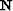andfor the multiples and the primaries, respectively, are needed. Therefore, models for the noise and the signal are necessary. For surface-related multiples, the multiple model may be provided by Surface-Related Multiple Prediction (SRMP) van Dedem (2002); Verschuur et al. (1992), which yields kinematically correct prestack model of the multiples, especially with 2-D data (see Appendixfor details). In 3-D, with marine acquisition geometry, the crossline offsets need to be heavily interpolated first. The interpolation results have then a direct impact on the prediction, as illustrated in Chapter.

Amplitude-wise, an accurate surface-related multiple model can be derived if (1) the source wavelet is known, (2) the surface source and receiver coverage is large and dense enough, and (3) all the terms of the series that model different orders of multiples are incorporated Verschuur et al. (1992). Often in practice, a single convolution of the input data (i.e., one term of the series) is usually performed, giving a multiple model with erroneous relative amplitudes for high-order multiples (see Appendix, equation ()). Dragoset and Jericevic (1998) detail the possible flaws introduced in the prediction due to acquisition parameters.

Because PEFs estimate patterns, incorrect relative amplitudes and kinematic errors can affect multiple suppression. However, as we shall see later, 3-D filters seem to cope better with noise modeling inadequacies.

Signal PEFs are more difficult to estimate since the primaries are usually unknown. As a possible solution to this problem, Spitz (1999) estimates a signal PEFby deconvolving a data PEF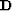, estimated from the data, by a noise PEF. With this process, Spitz assumes that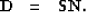(51)
I call equation () the Spitz approximation. Note that,, andare matrices for the combinations with the non-stationary PEFs Margrave (1998). These matrices are very sparse and are never formed in practice Claerbout and Fomel (2002). Equation () can be retrieved by considering a simple 1-D example using the Z-transform notations Claerbout (1976) for a data PEF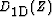,a signal PEF, and a noise PEF. Extension to more dimensions is straightforward using the helical boundary conditions Claerbout and Fomel (2002). Because PEFs have the inverse spectrum of the data from which they have been estimated Burg (1975), we have (omitting Z for clarity purposes)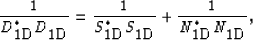(52)
where (*) is the complex conjugate. Equation () simply states that the spectrum of the data is equal to the spectrum of the noise plus the spectrum of the signal. Equation () can be written as follows:(53)
Now, because PEFs are important where they are small (i.e., where they attenuate seismic events), the denominator can be neglected: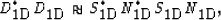(54)
which leads to the Spitz approximation in equation (). The PEFsandcan be easily estimated because the data vector and a noise model are often available. However, estimating the signal PEFs requires a potentially unstable non-stationary deconvolutionRickett (2001) in equation (). To avoid the deconvolution step, the noise PEFs are convolved with the data:(55)
whereis the result of the convolution. Estimating the PEFsforgives by definition of the PEFs Claerbout and Fomel (2002):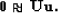(56)
Then, from the Spitz approximation in equation (), we have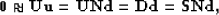(57)
and. Therefore, by convolving the data with the noise PEFs, signal PEFs consistent with the Spitz approximation can be computed. Again, an important assumption is that signal and noise are uncorrelated. The PEFs for the primaries () and the multiples () are estimated directly from the data and the model of the multiples. These filters approximate the multidimensional spectra of the noise and signal.Next: Multiple attenuation Up: Multiple attenuation: Theory and Previous: Filter estimation
Stanford Exploration Project
5/5/2005